Search

# Section 3: Results by Fields of Research Code

Section 3 provides a summary of ERA 2015 results and activity by aggregated four-digit FoR code. The four-digit codes that sit within each two-digit FoR are listed at the beginning of each discipline in this section.

Comparative information is presented for each discipline at the four-digit level and includes:

• Fields of Research overview with the aggregated four-digit data for each ERA indicator
• the distribution table and chart showing the number of UoEs per rating scale score (including the two- and four-digit ratings for that FoR)
• research outputs submitted by type (chart and table)
• research income by year-all categories (\$) (chart and table)
• staffing profile by academic level (chart and table)
• patent profile (where applicable) (table only)
• research commercialisation income by year (\$) (chart and table)

Following these summary charts, an overview table for each individual four-digit FoR code is presented as well as doughnut charts showing the distribution of output types and the number of UoEs by rating for that four-digit code.

Notes:

• Where an indicator does not apply to a particular discipline ‘-’ is shown. A ‘0’ represents that the indicator applies to the discipline, but no data was submitted.
• Percentages <1 not shown on doughnut charts for research outputs by type.
• Totals in the doughnut charts may not sum to 100% due to rounding.

## 01 Mathematical Sciences

Mathematical Sciences is comprised of the following four-digit codes:

• 0101 Pure Mathematics
• 0102 Applied Mathematics
• 0103 Numerical and Computational Mathematics
• 0104 Statistics
• 0105 Mathematical Physics
• 0199 Other Mathematical Sciences

18 out of 26 two-digit UoEs and 48 out of 59 four-digit UoEs assessed were rated above world standard

### FoR Overview

Mathematical Sciences (01) accounted for approximately two per cent of the research outputs submitted to ERA 2015. The most common research output type in Mathematical Sciences was journal articles. The staffing profile showed similar staffing levels and spread for Pure Mathematics (0101) and Applied Mathematics (0102) for academic levels B-E. These two four-digit codes showed the highest number of research outputs and research income. Research commercialisation income was particularly strong in Applied Mathematics (0102).

Indicator

No.

Research outputs

10,632.6

Research income

\$150,725,932

FTEs

922.6

Esteem count

147.2

Patents

0.2

Research commercialisation income

\$162,956

Rating

Distribution

Two-digit

Four-digit

5

7

23

4

11

25

3

8

11

2

0

0

1

0

0

Total

26

59

#### Number of UoEs per rating scale score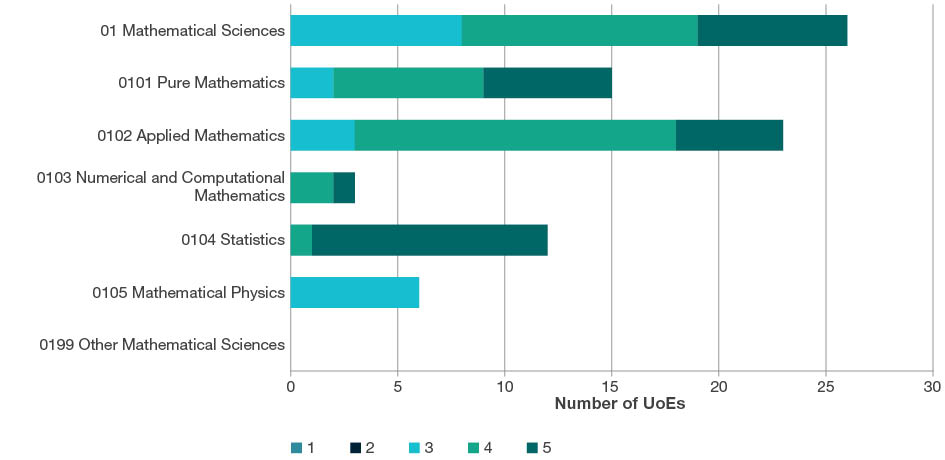Note: 01 Mathematical Sciences shows assessed two-digit UoEs only.

#### Research outputs submitted by type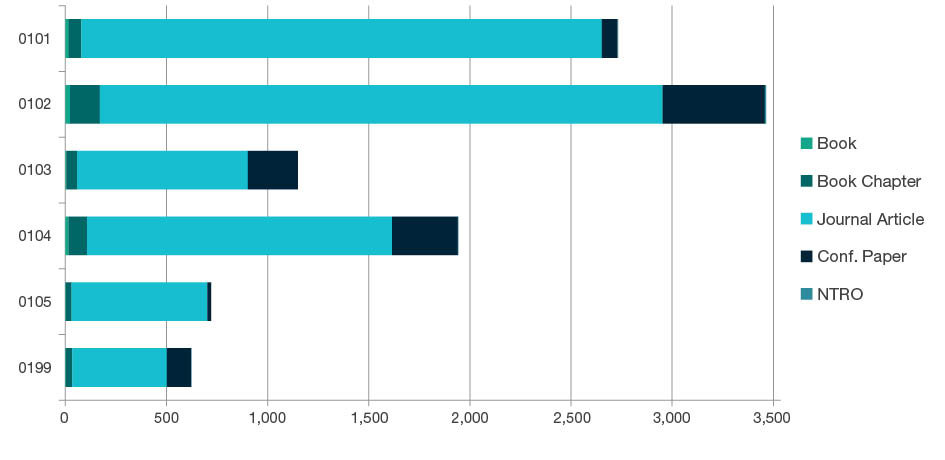FoR code

Book

Book Chapter

Journal Article

Conference Paper

NTRO

Total

0101 Pure Mathematics

17.7

61.6

2,573.3

78.5

1.0

2,732.0

0102 Applied Mathematics

23.9

146.6

2,782.0

506.3

5.0

3,463.8

0103 Numerical and Computational Mathematics

4.5

52.9

844.8

247.1

0.0

1,149.4

0104 Statistics

16.4

91.6

1,507.5

324.4

3.3

1,943.3

0105 Mathematical Physics

1.8

28.7

670.9

19.0

0.0

720.3

0199 Other Mathematical Sciences

2.0

32.3

467.7

119.8

2.0

623.8

Total

66.3

413.6

8,846.3

1,295.2

11.3

10,632.6

#### Research income by year – all categories (\$)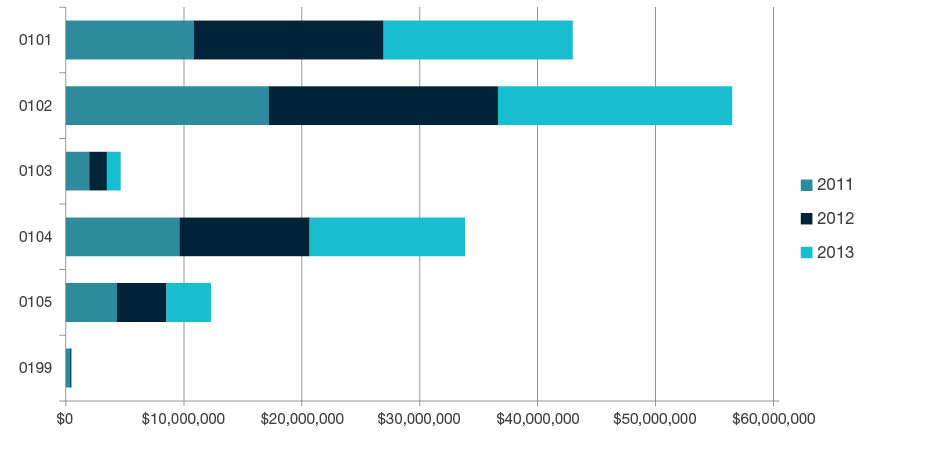FoR code

2011 (\$)

2012 (\$)

2013 (\$)

Total (\$)

0101 Pure Mathematics

10,879,050

16,063,937

16,021,651

42,964,638

0102 Applied Mathematics

17,232,269

19,421,834

19,838,752

56,492,855

0103 Numerical and Computational Mathematics

1,996,702

1,470,959

1,175,071

4,642,731

0104 Statistics

9,649,913

11,019,106

13,165,920

33,834,938

0105 Mathematical Physics

4,342,354

4,168,538

3,786,661

12,297,553

0199 Other Mathematical Sciences

366,382

53,676

73,158

493,216

Total

44,466,669

52,198,050

54,061,213

150,725,932

#### Staffing profile by academic level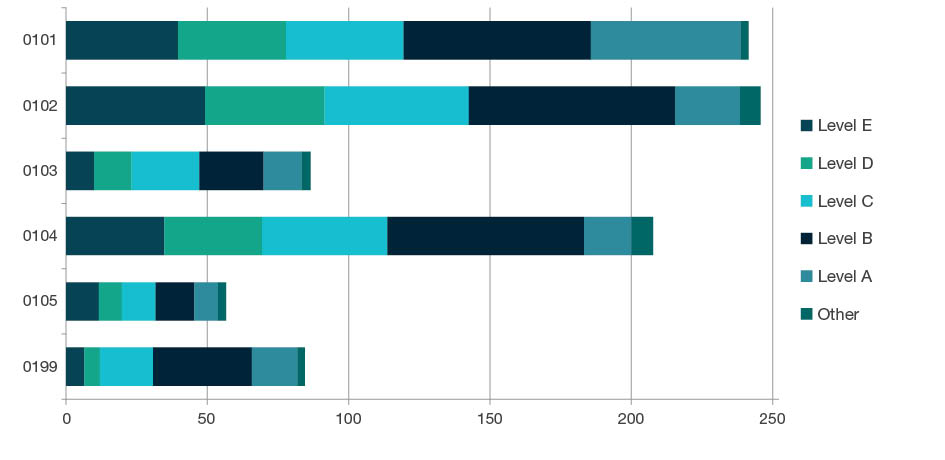FoR code

Level E

Level D

Level C

Level B

Level A

Other FTE

Total

0101 Pure Mathematics

39.7

38.2

41.5

66.4

52.9

2.8

241.5

0102 Applied Mathematics

49.3

42.2

51.0

72.9

23.0

7.2

245.7

0103 Numerical and Computational Mathematics

10.0

13.2

24.0

22.8

13.5

3.1

86.5

0104 Statistics

34.8

34.6

44.3

69.6

16.7

7.8

207.8

0105 Mathematical Physics

11.6

8.2

11.9

13.7

8.4

2.9

56.6

0199 Other Mathematical Sciences

6.5

5.5

18.8

35.0

16.1

2.7

84.5

Total

151.8

142.0

191.5

280.3

130.5

26.4

922.6

#### Patent profile

FoR code

Australia

USA

Europe

Japan

Other International

Total

0101 Pure Mathematics

0.0

0.0

0.0

0.0

0.0

0.0

0.0

0102 Applied Mathematics

0.0

0.0

0.0

0.0

0.2

0.0

0.2

0103 Numerical and Computational Mathematics

0.0

0.0

0.0

0.0

0.0

0.0

0.0

0104 Statistics

0.0

0.0

0.0

0.0

0.0

0.0

0.0

0105 Mathematical Physics

0.0

0.0

0.0

0.0

0.0

0.0

0.0

0199 Other Mathematical Sciences

0.0

0.0

0.0

0.0

0.0

0.0

0.0

Total

0.0

0.0

0.0

0.0

0.2

0.0

0.2

#### Research commercialisation income by year (\$)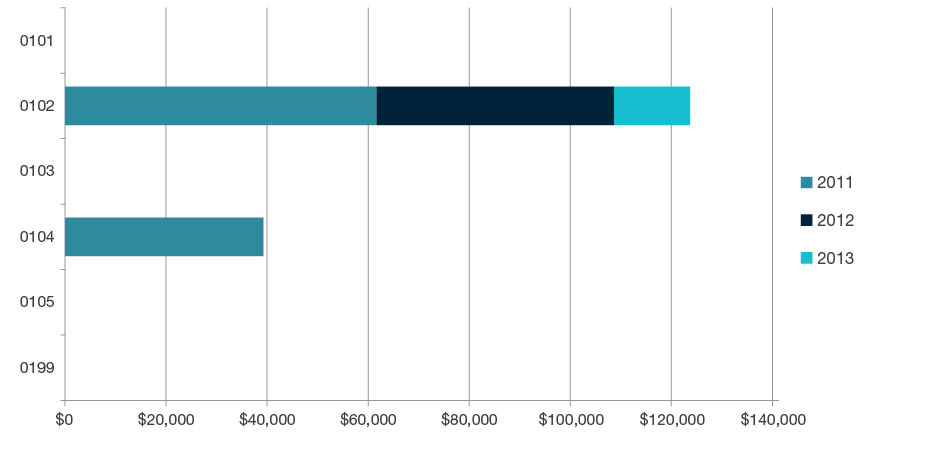FoR code

2011 (\$)

2012 (\$)

2013 (\$)

Total (\$)

0101 Pure Mathematics

0

0

0

0

0102 Applied Mathematics

61,674

47,025

14,980

123,679

0103 Numerical and Computational Mathematics

0

0

0

0

0104 Statistics

39,278

0

0

39,278

0105 Mathematical Physics

0

0

0

0

0199 Other Mathematical Sciences

0

0

0

0

Total

100,952

47,025

14,980

162,956